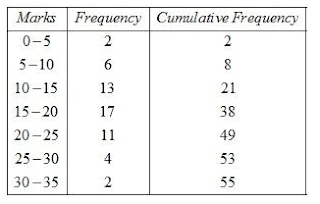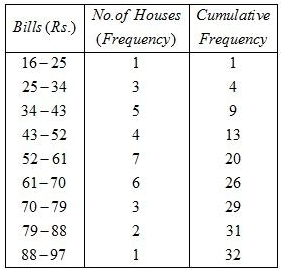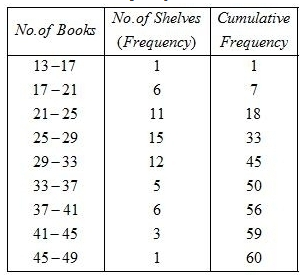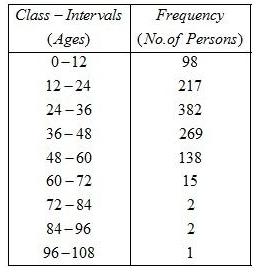#### Chapter 22 Tabular Representation of Statistical Data R.D. Sharma Solutions for Class 9th Exercise 22.2

Exercise 22.2

1. Define cumulative frequency distribution.

Solution

Cumulative frequency distribution is a table which displays the manner in which cumulative frequencies are distributed over various classes. In a grouped frequency distribution the cumulative frequency of a class is the total of all frequencies upto and including that particular class. Make sure that, for calculating cumulative frequencies, the classes should be written in ascending order.
For example, the following table gives the cumulative frequency distribution of marks scored by 55 students in a test :Cumulative frequency distributions are of two types, namely, less than and greater than or more than. For less than cumulative frequency distributions we add up the frequencies from the above and for greater than cumulative frequencies we add up the frequencies from below.

2. Explain the difference between a frequency distribution and a cumulative frequency distribution.

Solution

Frequency distribution displays the frequencies of the corresponding class-intervals. But, the cumulative frequency distribution displays the cumulative frequencies of the corresponding classes. For example, the following table gives the frequency and cumulative frequency distribution of marks scored by 55 students in a test:In a frequency distribution, the sum of all the frequencies is equal to the total number of observations. But, in the cumulative frequency distribution, the last cumulative frequency is same as the total number of observations. For example, in the above table the sum of all the frequencies is 55, which is same as the total number of students and the last cumulative frequency is 55, which is same as the total number of students.

3. The marks scored by 55 students in a test are given below:Prepare a cumulative frequency table.

Solution4. Following are the ages of 360 patients getting medical treatment in a hospital on a day:
 Age (in years): 10-20 20-30 30-40 40-50 50-60 60-70 No.of Patients: 90 50 60 80 50 30
Construct a cumulative frequency distribution.

Solution

The ages in years of 360 patients are given as5. The water bills (in rupees) of 32 houses in a certain street for the period 1.1.98 to 31.3.98 are given below:
56, 43, 32, 38, 56, 24, 68, 85, 52, 47, 35, 58, 63, 74, 27, 84, 69, 35, 44, 75, 55, 30, 54, 65, 45, 67, 95, 72, 43, 65, 35, 59.
Tabulate the data and present the data as a cumulative frequency table using 70-79 as one of the class intervals.

Solution

The minimum and maximum bills are 24 Rs. and 95 Rs.
The range 95-24 = 71 is.
Given that 70-79 is a class-interval. So, the class size 79 - 70 = 9 is.
Now calculate
Range/Class - size
= 71/9 = 7.89
Thus,the number of classes is 8
The cumulative frequency distribution is the following :6. The number of books in different shelves of a library are as follows:
30, 32, 28, 24, 20, 25, 38, 37, 40, 45, 16, 20
19, 24, 27, 30, 32, 34, 35, 42, 27, 28, 19, 34,
38, 39, 42, 29, 24, 27, 22, 29, 31, 19, 27, 25
28, 23, 24, 32, 34, 18, 27, 25, 37, 31, 24, 23,
43, 32, 28, 31, 24, 23, 26, 36, 32, 29, 28, 21
Prepare a cumulative frequencies distribution table using 45-49 as the last class-interval.

Solution

The minimum and maximum numbers of books in shelves are 16 and 45.
The range is 45 - 16 = 29.
Given that 45-49 is the last class-interval. So, the class size is 49 - 45 = 4.
Now calculate
Range/Class-size
= 29/4
= 7.25
Thus,the number of classes is 8.
The cumulative frequency distribution is the following :7. Given below are the cumulative frequencies showing the weights of 685 students of a school. Prepare a frequency distribution table.Solution

We make class intervals 0-25, 25-30, 30-35, 35-40, 40-45, 45-50, 50-55, 55-60, 60-65 and 65-70.
We will now find the frequencies of the different class-intervals from the cumulative frequency distribution table.
The frequency of the class 0-25 is 0.
The frequency of the class 25-30 is 24-0 = 24.
The frequency of the class 30-35 is 78-24 = 54
The frequency of the class 35-40 is 183-78 = 105.
The frequency of the class 40-45 is 294-183 = 111.
The frequency of the class 45-50 is 408-294 = 114.
The frequency of the class 50-55 is 543-408 = 135.
The frequency of the class 55-60 is 621-543 = 78.
The frequency of the class 60-65 is 674-621 = 53.
The frequency of the class 65-70 is 685-674 = 11.
Here is the cumulative frequency distribution table.

8. The following cumulative frequency distribution table shows the daily electricity consumption (in KW) of 40 factories in an industrial state:(i) Represent this as a frequency distribution table.
(ii) Prepare a cumulative frequency table.

Solution

We make class intervals 0-240, 240-270, 270-300, 300-330, 330-360, 360-390 and 390-420.
(i) We will now find the frequencies of the different class-intervals.
Just proceed reverse to you proceed to generate cumulative frequency
Here is the frequency distribution table.(ii) We are given the cumulative frequency table and we are asked to prepare new one.
So we will generate in some other fashion. Concentrate on frequency table and prepare the table. Here is the cumulative frequency distribution table.

9. Given below is a cumulative frequency distribution table showing the ages of people living in a locality:Prepare a frequency distribution table.

Solution

We make class intervals 0-12, 12-24, 24-36, 36-48, 48-60, 60-72, 72-84, 84-96 and 96-108.
We will now find the frequencies of the different class-intervals from the cumulative frequency distribution table.

The frequency of the class 0-12 is 1026−112 = 98
The frequency of the class 12-24 is1026−809 = 217
The frequency of the class 24-36 is 809−427 = 382
The frequency of the class 36-48 is 427−158 = 269
The frequency of the class 48-60 is 158−20 = 138
The frequency of the class 60-72 is 20−5 = 15
The frequency of the class 72-84 is 5−3 = 2
The frequency of the class 84-96 is 3−1 = 2
The frequency of the class 96-108 is 1−0 = 1
Here is the cumulative frequency distribution table.